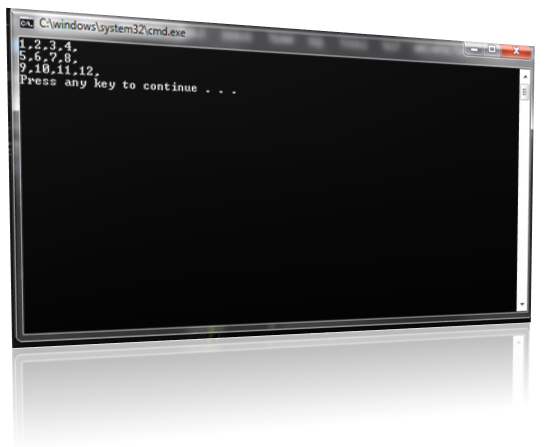# TWO DIMENSIONAL ARRAYS

## 1.1.   Two-Dimensional Arrays

C++ allows multidimensional arrays. The simplest form of the multidimensional array is the two-dimensional array. A two-dimensional array is, in essence, a list of one-dimensional arrays. To declare a two-dimensional integer array twod of size 10,20 you would write
 int twod;

Pay careful attention to the declaration. Unlike some other computer languages, which use commas to separate the array dimensions, C++ places each dimension in its own set of brackets. Similarly, to access point 3,5 of array twod, you would use twod. In the next example, a two-dimensional array is loaded with the numbers 1 through 12.
 // TWO DIMENSIONAL ARRAY.cpp : Defines the entry point for the console application. // #include "stdafx.h" #include using namespace std; int _tmain(int argc, _TCHAR* argv[]) {             int t, i, num;             for(t = 0; t < 3; ++t){                         for(i = 0; i < 4; ++i) {                                    num[t][i] = (t*4)+i+1;                                    cout << num[t][i] << ",";                         }                         cout << "\n";             }             return 0; }
In this example, num will have the value 1, num the value 2, num the value 3, and so on. The value of num will be 12.Win32 Console Application Window

Two-dimensional arrays are stored in a row-column matrix, where the first index indicates the row and the second indicates the column. This means that when array elements are accessed in the order in which they are actually stored in memory, the right index changes faster than the left. You should remember that storage for all array elements is determined at compile time. Also, the memory used to hold an array is required the entire time that the array is in existence. In the case of a two-dimensional array, you can use this formula to determine the number of bytes of memory that will be allocated:
 bytes = row × column × number of bytes in type
Therefore, assuming two-byte integers, an integer array with dimensions 10,5 would have 10 × 5 × 2 (or 100) bytes allocated.Matrix Table

Blogger Comment